﻿ 内分液结构调控竖直管间歇流型建模研究
«上一篇文章快速检索 高级检索

 力学学报2015, Vol. 47Issue (1): 61-70  DOI: 10.6052/0459-1879-14-2180

### 引用本文 [复制中英文]

[复制中文]
Ma Xiaolin, Xu Jinliang, Xie Jian, Xing Feng, Cheng Yongpan. MODEL RESEARCH ON MECHANISM OF MODULATED FLOW PATTERNS FOR VERTICAL UPFLOW[J]. Chinese Journal of Ship Research, 2015, 47(1): 61-70. DOI: 10.6052/0459-1879-14-218.
[复制英文]

### 文章历史

2014-07-24 收稿
2014-12-09 录用
2014–12–12 网络版发表

1 问题及物理模型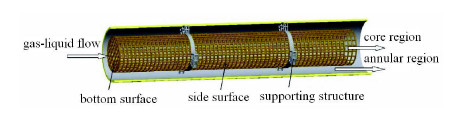图 1 内分液调控管示意图 Fig. 1 The proposed heat transfer tube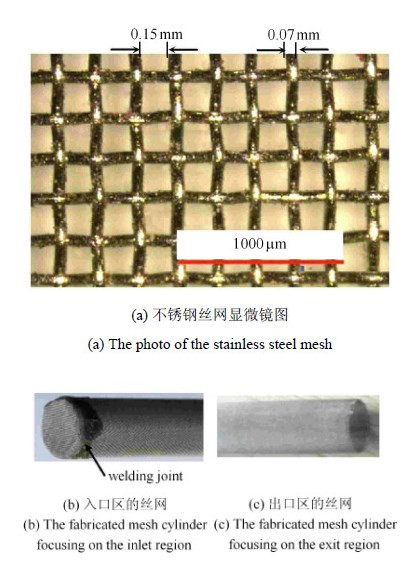图 2 流型调控管的丝网示意图 Fig. 2 The stainless steel mesh to fabricate the mesh cylinder

 ${\rm{d}} E = 4\sigma w {\rm{d}} s_2 - \sigma \pi D {\rm{d}} s_1$ (1)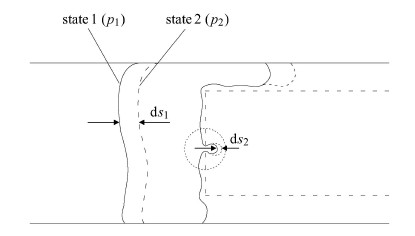图 3 大气泡试图穿过丝网孔示意图 Fig. 3 Large bubble surface energy required to prevent the bubble from entering the mesh cylinder

 $w^2 {\rm{d}} s_2 = \frac{\pi D^2}{4} {\rm{d}} s_1$ (2)

 ${\rm{d}} K = p_1 \frac{\pi D^2}{4} {\rm{d}} s_1 - p_2 w^2 {\rm{d}} s_2$ (3)

 $p_1 - p_2 = 4 \sigma \Big (\frac{1}{w} - \frac{1}{D} \Big)$ (4)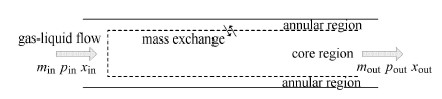图 4 丝网管一维物理模型示意图 Fig. 4 Sketch map of the physics picture
2 数学模型

2.1 质量方程

(1)质量守恒

 $m_a + m_c = {\rm const }$ (5)

(2)质量迁移

 $G_p = \rho \frac{\kappa }{\mu } \frac{{\rm{d}}elta p}{{\rm{d}}elta }$ (6)

(3)有效面积

 $r_w = \frac{L_l }{L_l + L_s } = 1 - \frac{L_s }{L_l + L_s }$ (7)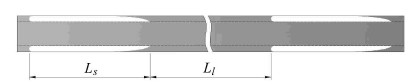图 5 润湿比定义的示意图 Fig. 5 Sketch map of wet ratio

 $r_w = 1 - \varepsilon$ (8)

 $A_{\rm eff} = \pi d l P r_w$ (9)

 $m_p = G_p A_{\rm eff}$ (10)

2.2 压降方程

(1)压降

 $\left( {\frac{{\rm{d}} p}{{\rm{d}} z}} \right)_{sp} = - \frac{2 f G^2}{\rho d} - \rho g$ (11)

 $\begin{array}{l} {\left( {\frac{{{\rm{d}}p}}{{{\rm{d}}z}}} \right)_{tp}} = \varphi _l^2{\left( {\frac{{{\rm{d}}p}}{{{\rm{d}}z}}} \right)_l} - \frac{{\rm{d}}}{{{\rm{d}}z}}\left( {\frac{{{G^2}{x^2}}}{{{\rho _g}\varepsilon }} + \frac{{{G^2}{{(1 - x)}^2}}}{{{\rho _l}(1 - \varepsilon )}}} \right) - \\ \qquad g\left[ {\varepsilon {\rho _g} + \left( {1 - \varepsilon } \right){\rho _l}} \right] \end{array}$ (12)

 $\varphi _l ^2 = \left( {1 + \frac{C}{X} + \frac{1}{X^2}} \right)$ (13)

 $X^2 = \frac{( {\rm{d}} p / {\rm{d}} z)_l }{( {\rm{d}} p / {\rm{d}} z)_g } = \frac{f_l }{f_g } \frac{\rho_g }{\rho _l } \Big (\frac{1 - x}{x} \Big)^2$ (14)

$C$是奇泽姆数，取值5$\sim$20.

(2)摩擦系数$f$

 $f = 16 / Re$ (15)

 $f = 0.079 / Re^{0.25}$ (16)

(3)空泡份额$\varepsilon$

 $\varepsilon = 0.833 \beta$ (17)

 $\varepsilon = \frac{J_g }{C_0 J + u_{gj} }$ (18)

 $C_0 = 1.2 ,\ \ u_{gj} = 1.53 \left[{\frac{g\sigma \left( {\rho _l - \rho _g }\right)}{\rho _l ^2}} \right]^{1 / 4}$ (19)

 $C_0 = 1.2 ,\ \ u_{gj} = 0.35 \left[{\frac{gD\left( {\rho _l - \rho _g }\right)}{\rho _l }} \right]^{1 / 2}$ (20)

3 模型求解与算法 3.1 预估$\!$-$\!$-$\!$修正量$S_{\rm ep}$与收敛判据

 $S_{\rm ep} = \frac{Q_a }{Q_c + Q_a }$ (21)

 $p_{a,{\rm out}} = p_{c,{\rm out}}$ (22)

3.2 边界条件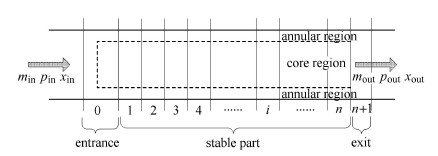图 6 流动区域网格划分图 Fig. 6 Control volumes of flow region

 $\left. \begin{array}{l} p_a = p_{a,1} ,m_a = m_{a,1} ,x_a = x_{a,1} , z = 0 \\ p_c = p_{c,1} ,m_c = m_{c,1} ,x_c = p_{c,1} , z = 0 \end{array}\!\! \right\}$ (23)

3.3 求解流程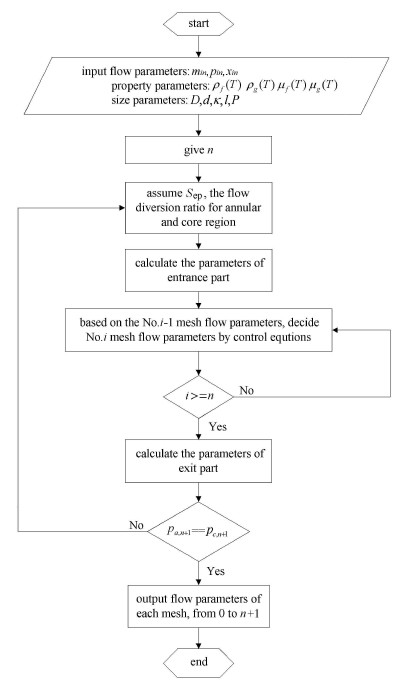图 7 模型求解流程图 Fig. 7 Flow chart of solving process
4 结果与讨论 4.1 模型计算与实验对比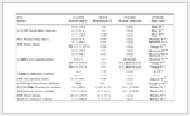表 1 工况表 Table 1 Work conditions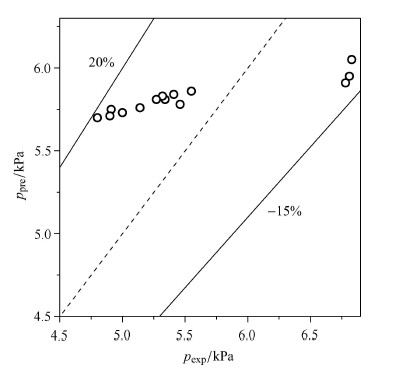图 8 压降实验值与模型预测值对比 Fig. 8 Comparison between experimental and predicted pressure drop
4.2 流动状态与气速、液速的关系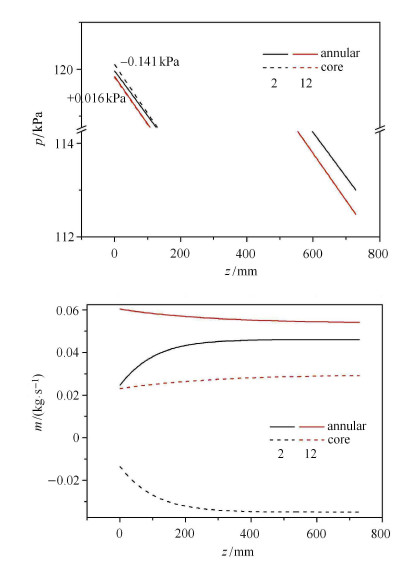图 9 两类工况流动参数沿程对比图 Fig. 9 Contrast of the second and the twelfth work condition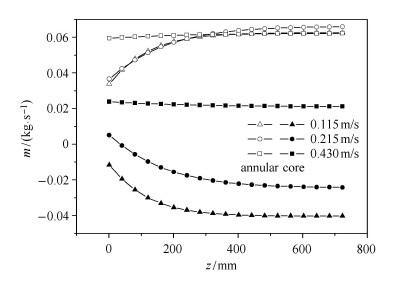图 10 气速0.115m/s，不同液速，工况5, 11, 13 Fig. 10 Gas velocity 0.115m/s, contrast of mass flow of different liquid velocity, cases 5, 11, 13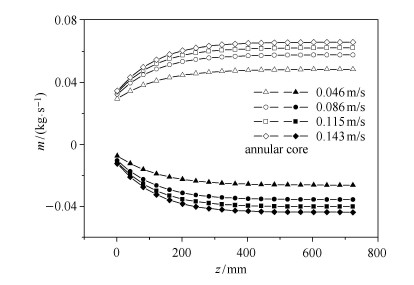图 11 液速0.115m/s，不同气速，工况3, 4, 5, 6 Fig. 11 Liquid velocity 0.115m/s, contrast of mass flow of different gas velocity, cases 3, 4, 5, 6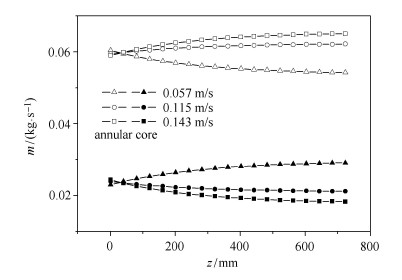图 12 液速0.430m/s，不同气速，工况12, 13, 14 Fig. 12 Liquid velocity 0.430m/s, contrast of mass flow of different gas velocity, cases 12, 13, 14

4.3 流动状态定量分析

$S_{\rm ep} >1$代表核心区一定出现竖直向下流(第1类工况)，为保证管道截面的净流量为竖直向上，环隙的竖直向上容积流量必然应大于管道截面净流量.$0 < S_{\rm ep} < 1$，则可能为第1类工况也可能为第2类工况.虽然入口处有竖直向上流穿过网底，但随后因渗流速度逐渐滞止为零进而转变为竖直向下流.这揭示了核心区出现速度滞止点的现象，与文献数值模拟速度滞止点现象相符.

 $S_{\rm ep} = f(J_l ,J_g )$ (24)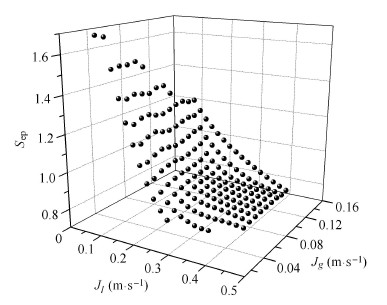图 13 采用三角立方插值加密数据点 Fig. 13 Data interpolation by cubic method

 $p(t) = {\rm{d}}sum_{i = 0}^n d_i N_{i,k} (t)$ (25)

 $d = (N^\tau WN)^{ - 1}N^\tau WP$ (26)

 ${\rm{d}}sum_{j = 1}^m \left[{p_j - {\rm{d}}sum_{i = 0}^n d_i N_{i,k} (t_j) } \right]^2 = \min$ (27)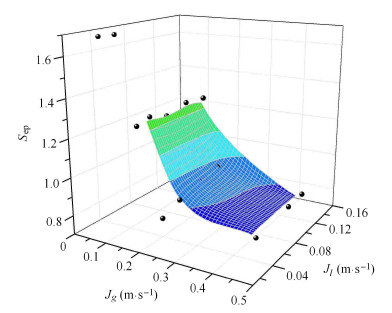图 14 拟合曲面与原始数据点 Fig. 14 Fitting surface and raw data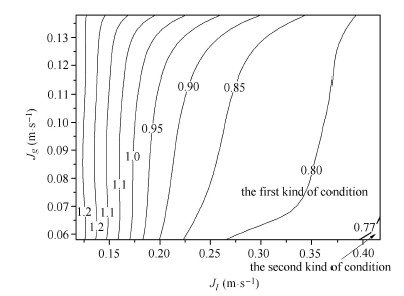图 15 Sep 等值线图 Fig. 15 Contour map of Sep
5 结 论

(1) 模型计算结果与实验误差在20%以内，吻合较好，可以说模型较好地解读了丝网管内的流动机理.

(2) 液速决定流动状态的大致轮廓，气速决定流动状态的细节.液速越高，沿程流量曲线位置越高，代表整体流量越大；而气速越高，沿程流量曲线越弯折，代表经丝网的渗透作用越剧烈.

(3) 当$Re_l < 6 937$时，核心区与环隙流体流动方向相反；$Re_l > 6 937$且$Re_g < 67$时，可能出现核心区与环隙流体流动方向相同现象，此时较低的气速会促使现象的出现.

  陈子乾, 何小荣, 王铁柱 等. 太阳能海水淡化系统经济性分析与研究. 工程热物理学报, 2012, 1: 002 (Chen Ziqian, He Xiaorong, Wang Tiezhu, et al. Economical Analysis of a solar Desalination System. Journal of Engineering Thermophysics, 2012, 1: 002 (in Chinese))  Graham D, Chato JC, Newell TA. Heat transfer and pressure drop during condensation of refrigerant 134a in an axially grooved tube. International Journal of Heat and Mass Transfer, 1998, 42(11): 1935-1944  Cavallini A, Del Col D, Doretti L, et al. Heat transfer and pressure drop during condensation of refrigerants inside horizontal enhanced tubes. International Journal of Refrigeration, 2000, 23(1): 4-25.  Miyara A, Otsubo Y. Condensation heat transfer of herringbone micro fin tubes. International Journal of Thermal Sciences, 2002, 41(7): 639-645.  Laohalertdecha S, Wongwises S. Condensation heat transfer and flow characteristics of R-134a flowing through corrugated tubes. International Journal of Heat and Mass Transfer, 2011, 54(11): 2673-2682  Han Y, Shikazono N, Kasagi N. The effect of liquid film evaporation on flow boiling heat transfer in a micro tube. International Journal of Heat and Mass Transfer, 2012, 55(4): 547-555.  Lips S, Meyer JP. Experimental study of convective condensation in an inclined smooth tube. Part I: Inclination effect on flow pattern and heat transfer coefficient. International Journal of Heat and Mass Transfer, 2012, 55(1): 395-404  Chen Hongxia, Xu Jinliang, Xie Jian, et al. Mudulated flow patterns for vertical upflows by the phase separation concept. Experimental Thermal and Fluid Science, 2014, 52: 297-307.  Chen Hongxia, Xu Jinliang, Li Zijin, et al. Stratified two-phase flow pattern modulation in a horizontal tube by the mesh pore cylinder surface. Applied Energy, 2013, 112: 1283-1290.  Chen Q, Xu J, Sun D, et al. Numerical simulation of the modulated flow pattern for vertical upflows by the phase separation concept. International Journal of Multiphase Flow, 2013, 56: 105-118.  Tsai JH, Lin L. Active microfluidic mixer and gas bubble filter driven by thermal bubble micropump. Sensors and Actuators A: Physical, 2002, 97-98: 665-671  Lawson KW, Lloyd DR. Membrane distillation. Journal of Membrane Science, 1997, 124(1): 1-25.  Handbook of Single-phase Convective Heat Transfer. New York: Wiley, 1987  Collier JG, Thome JR. Convective Boiling and Condensation. Oxford: University Press, 1994  Munson BR, Young DF, Okiishi TH. Fundamentals of Fluid Mechanics. New York, 1990  刘东,刘明侯,王亚青等. 带扰流小槽道内单相流动阻力特性实验. 力学学报, 2010, 42(6): 1006-1012 (Liu Dong, Liu Minghou, Wang Yaqing, et al. Experimental study on the frictional pressure drop of water flow in mini-channels with circular cylinder disturbed flow. Chinese Journal of Theoretical and Applied Mechanics, 2010, 42(6): 1006-1012 (in Chinese))  Armand AA. The Resistance During the Movement of a Two-Phase System in Horizontal Pipes. Atomic Energy Research Establishment, 1959  林宗虎, 王树众, 王栋. 气液两相流和沸腾传热. 西安: 西安交通大学出版社, 2003(Lin Zonghu, Wang Shuzhong, Wang Dong. Gas-liquid two-phase flow and boiling heat transfer. Xi'an: Xi'an Jiaotong University Press, 2003 (in Chinese))  Zuber N, Findlay JA. Average volumetric concentration in two-phase flow systems. Journal of Heat Transfer, 1965, 87(4): 453-468.  Ishii M. One-dimensional drift-flux model and constitutive equations for relative motion between phases in various two-phase flow regimes. Argonne National Lab., Ill.(USA), 1977  李岳生, 齐东旭. 样条函数方法. 北京: 科学出版社, 1979 (Li Yuesheng, Qi Dongxu. Spline Function Method. Beijing: Science Press, 1979 (in Chinese))
MODEL RESEARCH ON MECHANISM OF MODULATED FLOW PATTERNS FOR VERTICAL UPFLOW
Ma Xiaolin, Xu Jinliang, Xie Jian, Xing Feng, Cheng Yongpan
The Key Laboratory on Multiphase Flow and Heat Transfer, North China Electric Power University, Beijing 102206, China
Fund: The project was supported by the National Basic Research Program of China (2011CB710703) and the National Natural Science Foundation of China of International cooperation project (51210011).
Abstract: Due to the fact that micropores prevent gas while pump liquid according to their capillary force characteristics, the tube with mesh construction inside is proposed to modulate flow patterns and enhance heat transfer. Based on Lockhart-Martinelli's separated flow model and Zuber-Findlay's drift model, one-dimensional mathematic model is built to describe the flow dynamic behaviors in vertical flow pattern modulated tube. The experimental conditions are solved by mathematic model and the relative errors between model predicted and experimental results are no more than 20%. It is noted that liquid velocity plays a more important role on flow phenomena than gas velocity while gas velocity influences the degree of penetration through the mesh. Based on qualitative analysis, cubic interpolation and least squares B-spline fitting are used to obtain the quantitative function relationship between flow phenomena and superficial velocity. It comes to the conclusion that when Rel <6937, there must be the first kind of condition, and when Rel > 6937 with Reg <67, there can be the second kind of condition which is more likely to present at lower gas velocity. The mathematic model and quantitative function can contribute to the optimal design of flow pattern modulated tube.
Key words: flow pattern modulation    capillary force    vertical    separated flow model    least squares